How to Get the List of File Names From a Folder

This post will teach you how to get the list of file names in a given directory to a worksheet in Excel. You will learn that how to use Excel to view Files and Folders in your worksheet by get the list of file names with different ways, such as: Excel VBA Macro, or FILES function.

If you want to get the list of file names from a folder that contain hundreds of files in it. You may be think the simplest way is that selecting the file and copying its name, then paste the file name in a cell in your worksheet, then repeat the above steps. If you follow this way to do it, you will take a long time to complete it. So it is a simple way, but it is also a huge waste of time. Do we have some quick way to achieve the same result? Of course yes, the below will talk two ways to copy the list of file names from a folder into worksheet quickly.

Method 1: Using FILES function to get list of file names

You can use the FILES function to get the list of file names from a directory, and this function is only works in name Defined Names. And then you can use a combination of the IFERROR function, the INDEX function and the Row function to create an excel formula. Let’s see the below steps:

1# Assuming that you want to get the list of file names from a directory, such as: C:\Users\devops\Tracing\WPPMedia\*, and enter this directory path into Cell B1.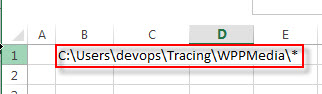2# On the FORMULAS Tab, click Define Name command under Defined Names group, then select Define Names… from the drop-down menu list.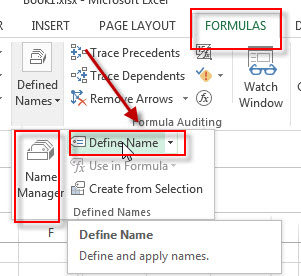3# the New Name window will appear. Set a name in the Name box, such as: FileNameList, type the following formula in the Refers to box.

=FILES(Sheet7!\$B\$1)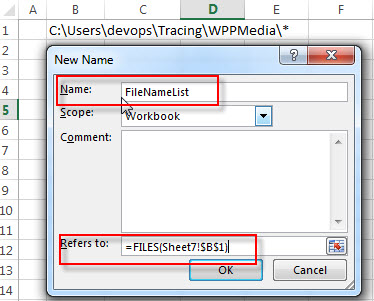4# enter the following formula in the Cell B3, then drag the AutoFill Handle down to others cells to apply this formula.

=IFERROR(INDEX(FileNameList,ROW()-2)," ")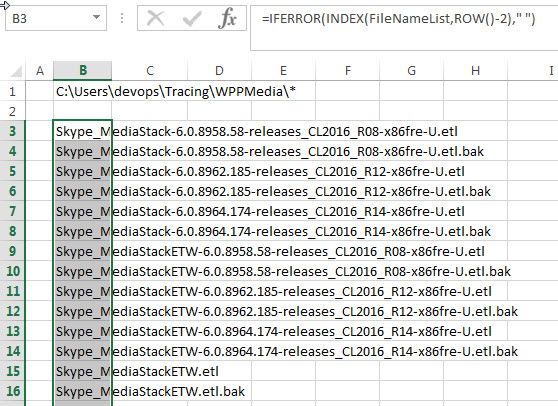You will see that all file names from the specified folder are listed in your worksheet.

Method 2: Using File browser and web browser to get list of file names

If you want to use this method to get the list of file name from a directory, you need to install one web browser, such as: Firefox, Chrome or IE. Then do it follow steps:

1# Move to the destination folder or directory on the Windows File Explorer, then copy the path of that directory. Such as: C:\Users\devops\Tracing\WPPMedia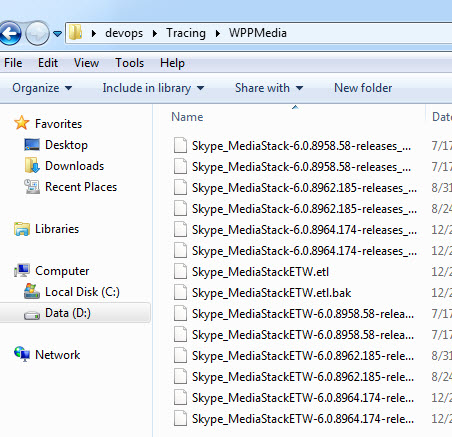2# open any web browser installed in your computer and paste the copied path in the address bar, then press Enter key.

3# you will see that it will add prefix file:/// at the beginning of the path in the address bar. And the file name list will be shown in the web page.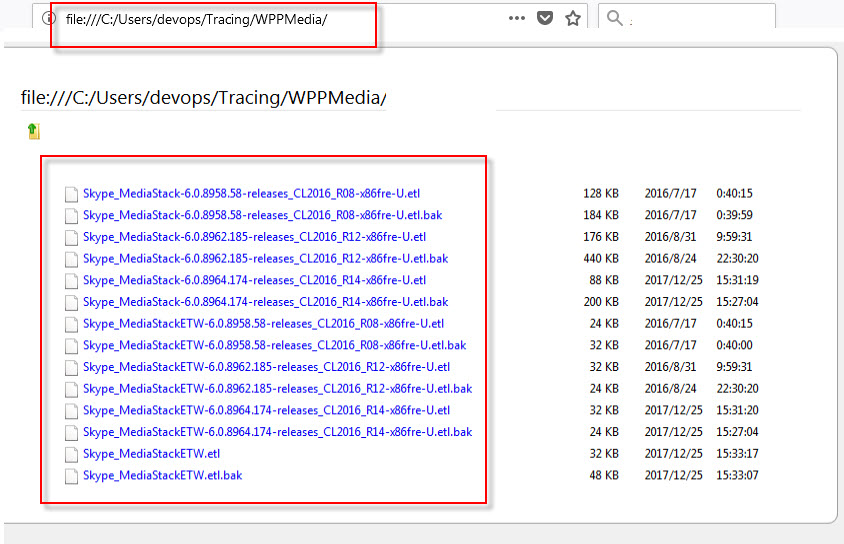4# press Ctrl + A shortcut to select all of file names in the web page and then press Ctrl +C to copy the selected content.

5# open your worksheet, then press Ctrl + V to paste all file names into your worksheet.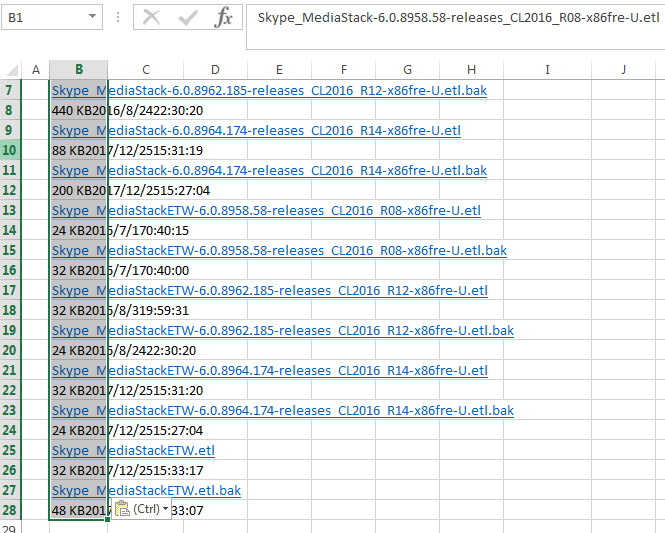Or you can save the web page in the Step 3 as the offline copy. Just press Ctrl + S or right-click on the web page, and then select Save Page As to save that web page.

Open the saved web page from the web browser, and copy its web address. Then click From WEB command under Get External Data group on the DATA tab in your worksheet.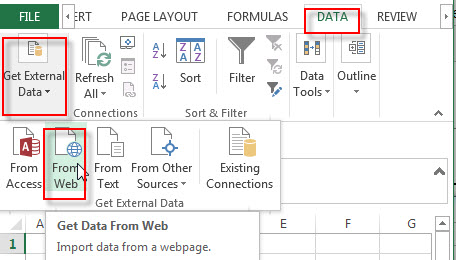Paste the web address in Address box, then click Go button. Then click Import button. You will see that all files and folders details are imported to your worksheet.

Method 3: Using Excel VBA macro to get list of file names

You can write an excel VBA macro code to get the list of file names from a specified directory,

1# click on “Visual Basic” command under DEVELOPER Tab.2# then the “Visual Basic Editor” window will appear.

3# click “Insert” ->”Module” to create a new module4# paste the below VBA code into the code window. Then clicking “Save” button.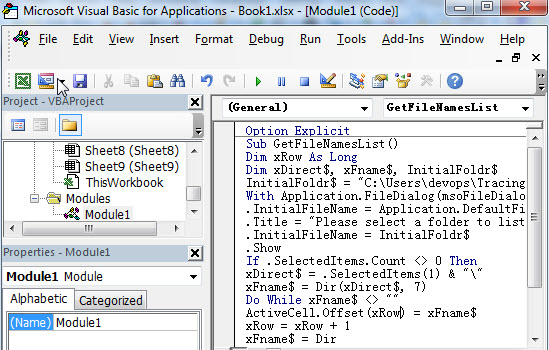Option Explicit
Sub GetFileNames()
Dim xRow As Long
Dim xDirect\$, xFname\$, InitialFoldr\$
InitialFoldr\$ = "C:\"
With Application.FileDialog(msoFileDialogFolderPicker)
.InitialFileName = Application.DefaultFilePath & "\"
.Title = "Please select a folder to list Files from"
.InitialFileName = InitialFoldr\$
.Show
If .SelectedItems.Count <> 0 Then
xDirect\$ = .SelectedItems(1) & "\"
xFname\$ = Dir(xDirect\$, 7)
Do While xFname\$ <> ""
ActiveCell.Offset(xRow) = xFname\$
xRow = xRow + 1
xFname\$ = Dir
Loop
End If
End With
End Sub

5# back to the current worksheet, then run the above excel macro.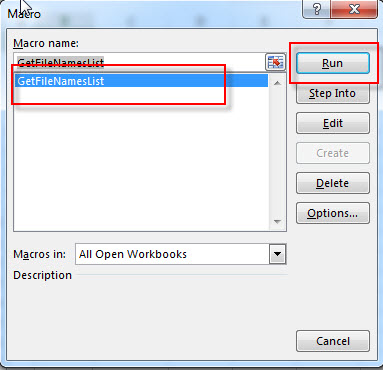6# select a folder that you want to get all names, then click OK button.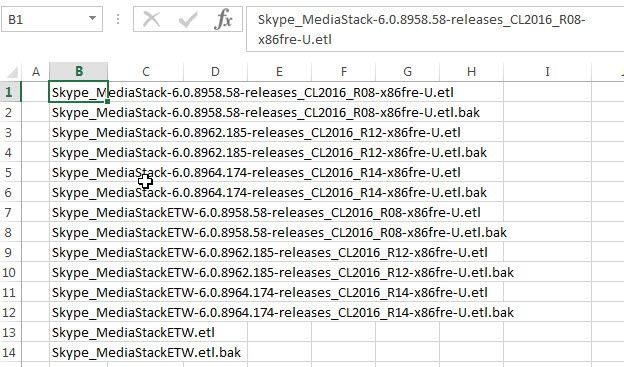Related Functions

• Excel IFERROR function
The Excel IFERROR function returns an alternate value you specify if a formula results in an error, or returns the result of the formula.The syntax of the IFERROR function is as below:= IFERROR (value, value_if_error)…
• Excel INDEX function
The Excel INDEX function returns a value from a table based on the index (row number and column number)The INDEX function is a build-in function in Microsoft Excel and it is categorized as a Lookup and Reference Function.The syntax of the INDEX function is as below:= INDEX (array, row_num,[column_num])…
• Excel ROW function
The Excel ROW function returns the row number of a cell reference.The ROW function is a build-in function in Microsoft Excel and it is categorized as a Lookup and Reference Function.The syntax of the ROW function is as below:= ROW ([reference])….
Related Posts

VBA Macro For VLOOKUP From Another Sheet

In the previous post, you should know that how to fix or remove the #N/A error when using VLOOKUP formula to lookup value from another sheet. And this post will show you how to use VBA code to vlookup data ...

How To Insert Comments in Protected Worksheet in Excel

This post will show you how to allow comments in a protected worksheet in Excel. You can easily to insert comments into cells in a normal worksheet in Excel, but if want to insert a comment in a worksheet that ...

How To Convert Text to Upper Cases(Using VBA) in Excel

This post will show you how to switch from lower case to upper case in Excel. and I am going to show you two different ways of converting text to upper cases using formula or VBA macro in Excel 2013,Excel ...

How To Hide Every Other Row in Excel (Using VBA)

This post will show you how to hide alternate rows or columns in Excel or how to hide every third, fourth, fifth row or column in Excel. If you want to hide every other row in your current worksheet, how ...

How to Disable the Save As Prompt in Excel

This post will show you how to use a VBA Macro to save an Excel file and overwrite any existing file without a prompt so that you are going to get the little window that says file already exists do ...

How to Count Cells that Contain even or odd numbers in Excel

This post will guide you how to count the number of cells that contain odd or even numbers within a range of cells using a formula in Excel 2013/2016.How do I count cells that contain odd numbers through the use ...

How to Count Cells that Contain negative Numbers in Excel

This post will guide you how to count the number of cells that contain negative numbers within a range of cells using a formula in Excel 2013/2016.You can count the number of negative numbers in your data using easy functions ...

How to Count Cells Are Not Blank or Empty in Excel

This post will guide you how to count cells that are not blank or empty in a given range cells using a formula in Excel 2013/2016.How do I count the number of cells that are not blank in a particular ...

How to Count Cells Not equal to X or Y in Excel

This post will guide you how to count the number of cells not equal to criteria X or Y in a given range cells using a formula in Excel 2013/2016.You can easily to count cells equal to or not equal ...

How to Count Cells Less Than a Specific Value in Excel

This post will guide you how to count the number of cells less than a particular numeric value in a given range cells using a formula in Excel 2013/2016. How do I count cells that are less than a specific ...

Sidebar